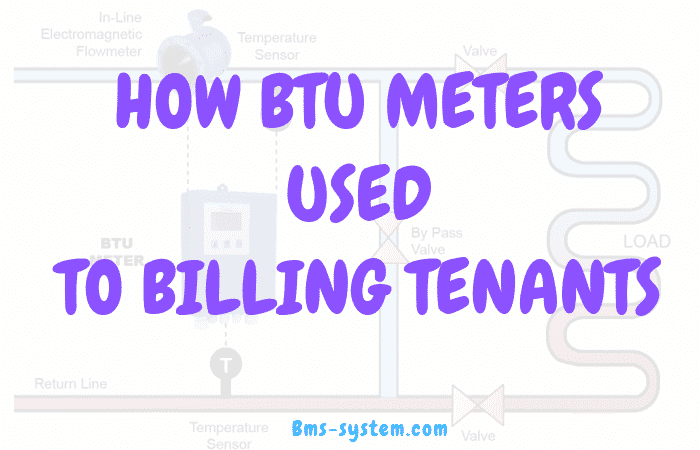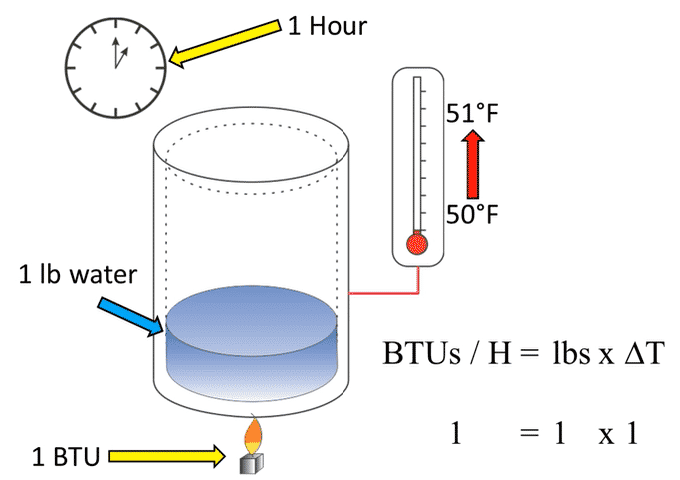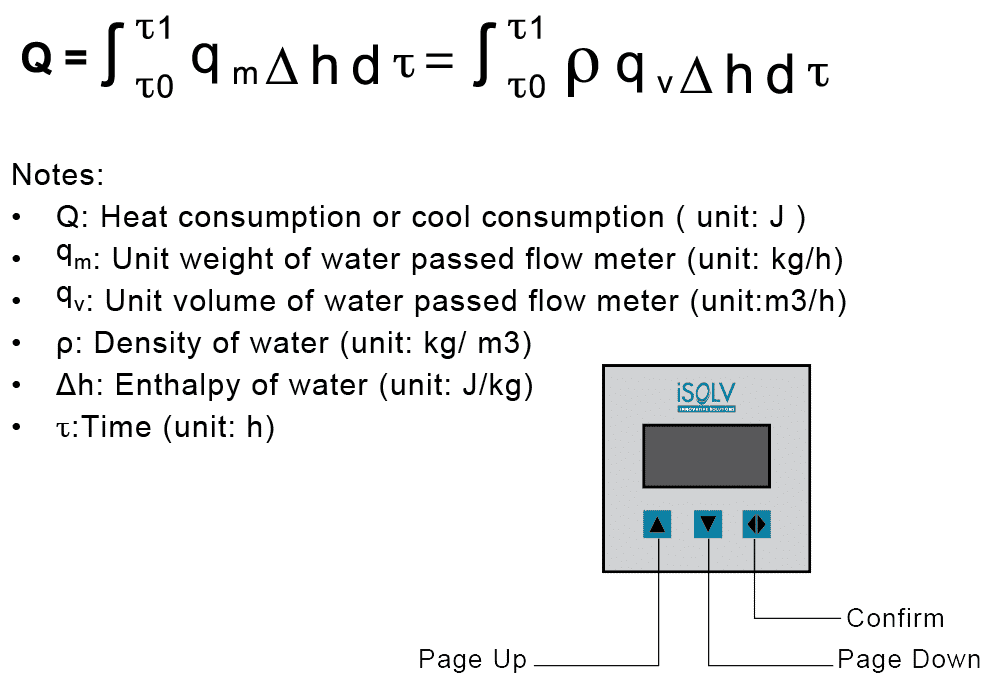# What is BTU Meter and how to calculate energy consumption for billing tenants

## What is BTU?

Before we proceed to learn what is BTU meter, let us see what is exactly BTU or British thermal unit.BTU is the amount of energy required to heat a single pound(lb) of water by one degree Fahrenheit.Let us assume the flame will burn for exactly one hour and will give out exactly one BTU.
Before starting the process, water temperature is at 50 degrees Fahrenheit, after one hour of one BTU(thermal heat energy) process will increase water temperature by 51-degree Fahrenheit.
the mathematical expression of the process is

BTU/Hr=lbs x Delta T

Delta T is the amount of temperature difference before and after the process, So here

BTU/Hr is 1 (which is considered as a flame)

Mass of water in lbs is 1

and delta T is 51-50=1 degree-Fahrenheit

let us see the second example to find the delta T for the given BTULet us assume that mass of water is 4 lbs and given BTU (flame or heat energy) is 2 and  find the delta T ie, Temperature increased of water after one hour

BTU/Hr=lbs x Delta T

now Delta T = BTU/lbs

Here the temperature increased is 0.5 degree-Fahrenheit.
Have a look at this video

## How to calculate the energy consumption by BTU Meters

BTU Measurement System is used to measure individual energy consumption in any liquid heating/cooling systems. This system is also used to measure the performance of the energy-saving system or the loss of efficiency which is directly tied to the loss of revenue.

BTU Meter is supplied complete with flowmeter and temperature probes.

The system real-time detect the temperature of supply and return pipe. It also monitors the instantaneous flow rate according to the Heat Exchange of Thermodynamics principle. The BTU meter automatically integrates energy consumption and transfers it to the computer. The consumption volume can be checked by a tenant or operator with no worries. It provides real-time information such as instantaneous temperature, flow rate, energy consumption, and others.

## How to Install BTU Meter

Careful attention to the site selection for the system components will help the installers with the initial installation, reduce start-up problems, and make future maintenance easier. For example, do not install the flow meter where it will be difficult for personnel to perform periodic maintenance.

• The Main Unit

Find an easily accessible location where wire connections can be made and meter readings can be taken from floor level. Mount the System enclosure on a vibration-free surface.
Avoid locations such as the plenum of a fan coil, heat exchanger or any housing that may contain electric motors or other strong sources of electrical interference.

• The Flow Meter

When properly installed, the flow meter will only measure flow associated with that portion of the piping system for which the energy measurement is being made. The flow meter may be
installed in either the supply or return line. Choose the location with the longest straight run of unobstructed pipe. Please refer to the flow meter installation manual for specific information
regarding the straight run requirements for the flow meter used with this SYSTEM BTU Meter.

• The Temperature Sensors

The two temperature sensors must be located in such a manner as to accurately measure only the temperature of the supply line entering and the return line leaving the portion of the piping system for which the energy measurement is being made.

If possible, find an easily accessible location where wire connections can be made from floor level. This will facilitate any future service. Place the temperature sensors away from strong sources of electrical noise that might affect the performance of the sensors.

One temperature sensor thermowell will need to be placed in the same pipe with the flow meter. It should be located on the downstream side of the flow meter. The downstream distance between the thermowell and flow meter should be at least five pipe diameters, leaving enough clearance to remove either sensor from the pipe without interference from the other sensor.to download onicon flowmeter installation and operation guide for the detailed study

## Operation of BTU Meter

When the water or other liquid passes through the pipeline, the flow meter measures the instantaneous flow rate and sends it to the BTU meter then the temperature sensor measures the return pipe temperature and supply pipe temperature and also sends it to BTU meter.

According to the above formula, BTU meter integrates the cooling or heat consumption. If T1>T2, integrates cooling consumption, otherwise integrates heat consumption. Finally, BTU meter stores the data and displays on LCD and can connect to existing BMS system.

### Measuring principles

Prerequisites knowledge to understand below calculation

BTU meter calculates energy consumption by flow rate and temperature difference, the formula is:No need to worry about this complex calculation, from the above parameters of supply, return temperature and flow rate, BTU meter calculates the heating/cooling energy consumption and send it through any building networking protocols like BACnet, Modbus etc to the existing BMS system or HMI display to the owner and this data can be used to bill the tenants.

And check out some of the manufactures of BTU Meters around worldwide.

Now let us see

### How to calculate electrical costs for cooling and power consumption

A BTU is approximately a third of a watt-hour. 1000 BTU/h is approximately 293W.  Kilowatt-hour (kWh) is most commonly known as a billing unit for energy delivered to consumers by electric utilities.

Cooling or heating cost calculation

For calculating the heating/cooling cost to the tenants,  use the formula where we translate the BTU/h to Watts needed so we get the correct costs associated with cooling or heating of the tenants.Note: Normally in the building, they will add extra cost like operating Cost (electricity, maintenance, meter reading, invoicing tenants) with energy consumption.

Now let us see,

### Method-1 of Billing tenants

Method 1
(Not Effective way but cheaper installation cost)

Calculate the total BTU’s consumed by the central chiller from the plant on where BTU Meter installed and divide it by entire space on the building in square feet and bill each customer based on how much space and time they used.

But this method not practical due to heat load calculation will not be considered based on the space only, but also another factor will affect the load calculation like

• Space the room size.
• Based on the window size, installation and shading level.
• The number of tenants occupied.
• Based on the heat generated by the other equipment like workstation, machinery etc.
• The heat generated by lighting.

So there is possible for a tenant to consume more heat load in a small space rather than the big space.

### Method-2 of Billing tenants

(Effective method but higher installation cost)

Install the BTU meter on each unit of the room the chiller or other equipment serving, so that we can calculate exact usage BTU’s used by the tenant and can be billed customer effectively.

### 13 thoughts on “What is BTU Meter and how to calculate energy consumption for billing tenants”

1.make on separate on flow meter also

2.I have fixed a BTU meter for one of the tenant and on what basis I have to charge them on a monthly basis.
Brand- Onicon
There are many readings like volume rate energy total,running hours volume total and energy rate.
to simplify and to convince the tenant which is the simplest method can I adopt.

3.However, check out this forum for understanding the billing tenant using BTU Meter

and approach Onicon support team to get rights answers.

Check out this also

ALLOCATION METHOD BASED ON ENERGY METER READING AND SQUARE FOOTAGE OF APARTMENT

This sample allocation method is for six tenants and is based on Energy Meter Readings and Area of the tenant’s apartment (base cost).

The apartments in this example have three different square foot areas. 50% of the total energy cost will be allocated based on the square foot area of each apartment and 50% will be allocated on the energy meter readings.

1) Monthly Cost of Energy (oil, gas, electricity) = \$480
2) Operating Cost (electricity, maintenance, meter reading, invoicing tenants) = \$56
3) Total Energy Cost = \$536

DISTRIBUTION OF ENERGY COST

Size of Apartment = 50%

4) Cost Allocation of Square Foot Area (amount allocated) = \$268
Total Square Footage (all tenants) = 3350
Cost per Square Foot (3350 @ 8?) = \$268

Tenant A (500 sq. ft. @ 8?) = \$40
Tenant B (600 sq. ft. @ 8?) = \$48
Tenant C (550 sq. ft. @ 8?) = \$44
Tenant D (550 sq. ft. @ 8?) = \$44
Tenant E (550 sq. ft. @ 8?) = \$44
Tenant F (600 sq. ft. @ 8?) = \$48

Total = \$268

5) Cost Allocation on Meter Reading (amount allocated) = \$268
Total Energy Units Used (all tenants) = 6700
Cost per Energy Unit (6700 @ 4?) = \$268

Tenant A Energy Units (1100 @ 4?) = \$44
Tenant B Energy Units (1300 @ 4?) = \$52
Tenant C Energy Units (800 @ 4?) = \$32
Tenant D Energy Units (1000 @ 4?) = \$40
Tenant E Energy Units (1600 @ 4?) = \$64
Tenant F Energy Units (900 @ 4?) = \$36

Total Energy Units (6700 @ 4?) = \$268

6) Individual Billing to Tenants

Tenant A Square Footage Base Cost \$40 + Energy Units Used (1100 @ 4?) = \$44, Total = \$84
Tenant B Square Footage Base Cost \$48 + Energy Units Used (1300 @ 4?) = \$52, Total =100
Tenant C Square Footage Base Cost \$44 + Energy Units Used (800 @ 4?) = \$32, Total =76
Tenant D Square Footage Base Cost \$44 + Energy Units Used (1000 @ 4?) = \$40, Total = \$84
Tenant E Square Footage Base Cost \$44 + Energy Units Used (1600 @ 4?) = \$64, Total = \$108
Tenant F Square Footage Base Cost \$48 + Energy Units Used (900 @ 4?) = \$36, Total = \$84

Total = \$536

https://www.istec-corp.com/products/btu-energy-meters-overview.htm

4.This website is usefull

1.Thanks chandru

5.Sir,
One of the btu meter of tenant is showing kW reading 300 or more than 300 .It is used for hvac system. Inlet and outlet temp sensor showing reading like 14deg.& ,32deg. Is the problem in btu meter or in temp sensor?

1.Hi Mahmoud,
Problem also causes due to may be other reasons like flow sensors.

Yes, there is more possibilities of temp sensor too.

BTU meter is just doing calculations based on thr input values.

Even I have faced problem recently due to temperature sensors faulty.

1.Hi Hamza, Im working for Flotech Controls. and it appear theres a broken link to our website. Please contact me at [email protected] and should resend you the latest url for the updated page. Thanks!

6.Amr elafifi( HVC engineer)

The cost equation is right for heating load only, but for cooling load we have to multiple by the C O P which equal (cooling load divided by power consumption).

7. Pingback: BTU Meter Manufacturers | BMS System

8.AHU is connected with 3 btu meters and 3 bypass line. Will there be cooling for AHU? Is there any problem by connecting them like that to AHU.

9.btu meter showing 0.1 mwh so how to convert in KWH

1.Check the display settings configuration in btu meter

Scroll to Top